Maths-
General
Easy

Question

# The line passing through the points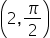, (3,0) is

##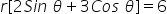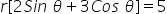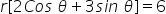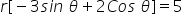Hint:

## The correct answer is:### x = a, where an is the x-coordinate of each point on the line, is the equation of a straight line that is vertical or parallel to the Y-axis.For instance, the equation of the line passing through the point (2,3) and parallel to the X-axis is y= 3.The line that is perpendicular to the Y-axis and contains the point (3,4) also has the equation x = 3.Here we have given the points, (3,0).Now, the equation of line joining the points (r1​,θ1​) and (r2​,θ2​) is given by:So here we used the concept of the equation of the line passing through two points. Here we also used the trigonometric terms to find the answer using the formulas. So the final solution is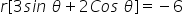.#### With Turito Foundation.#### Get an Expert Advice From Turito.